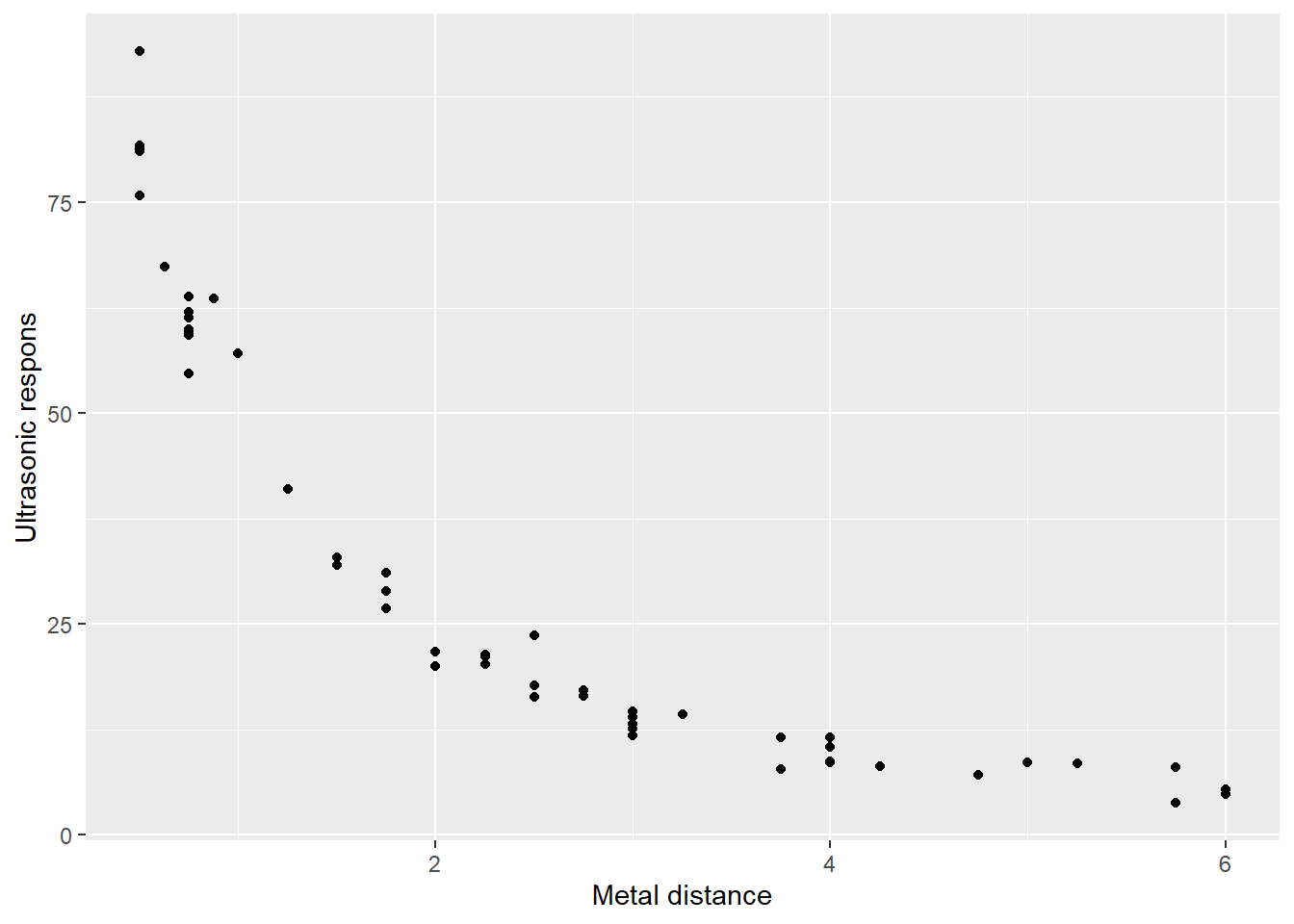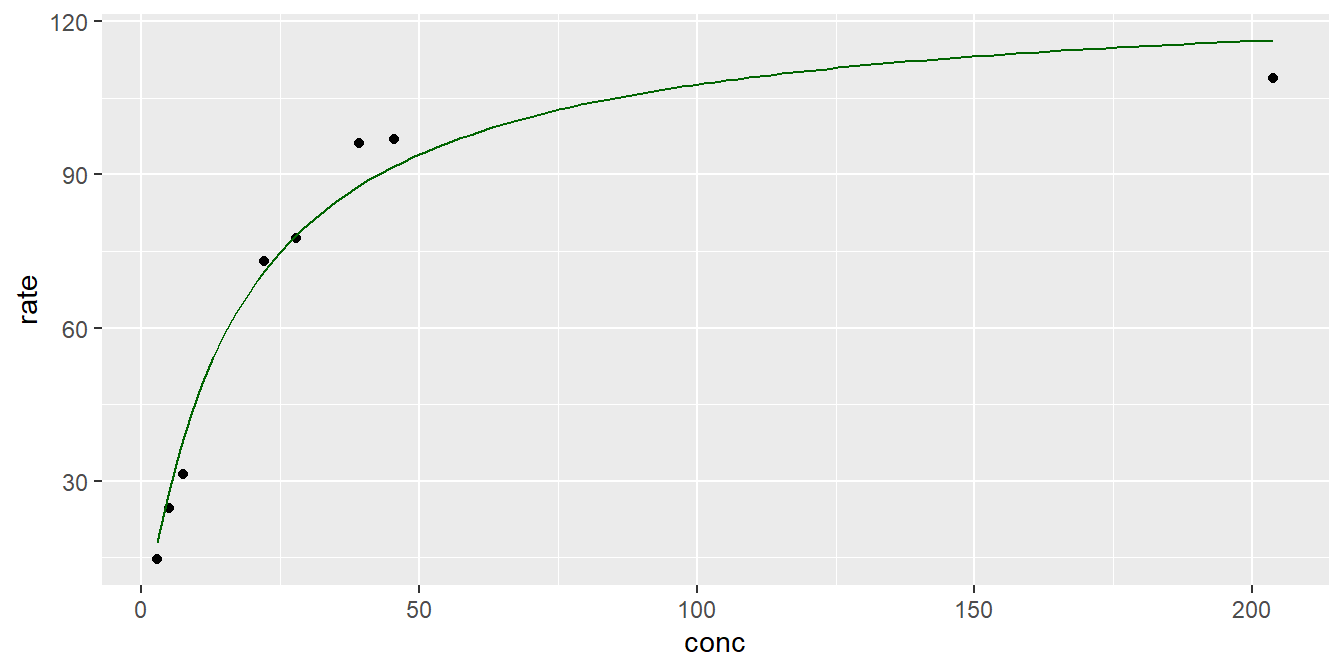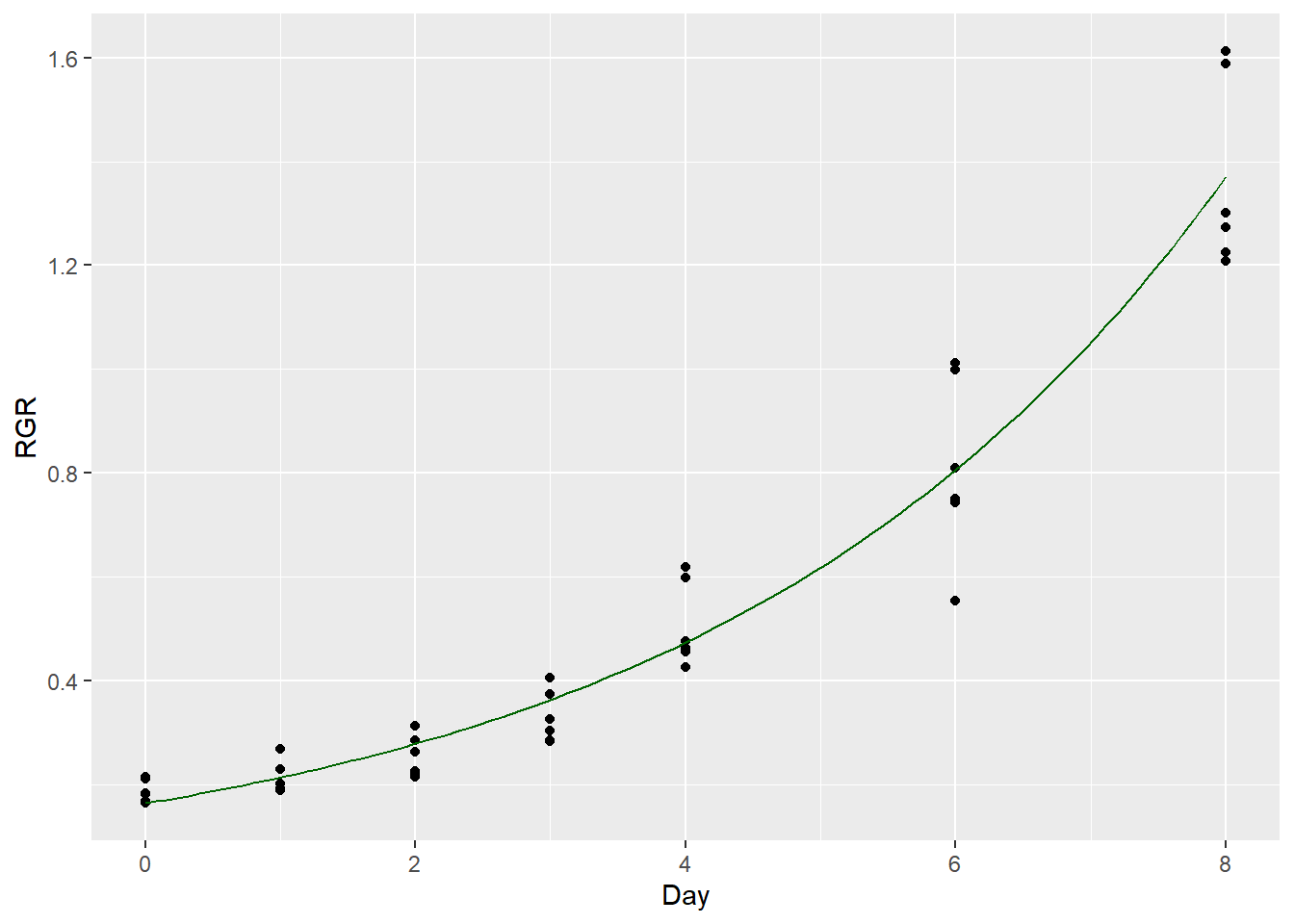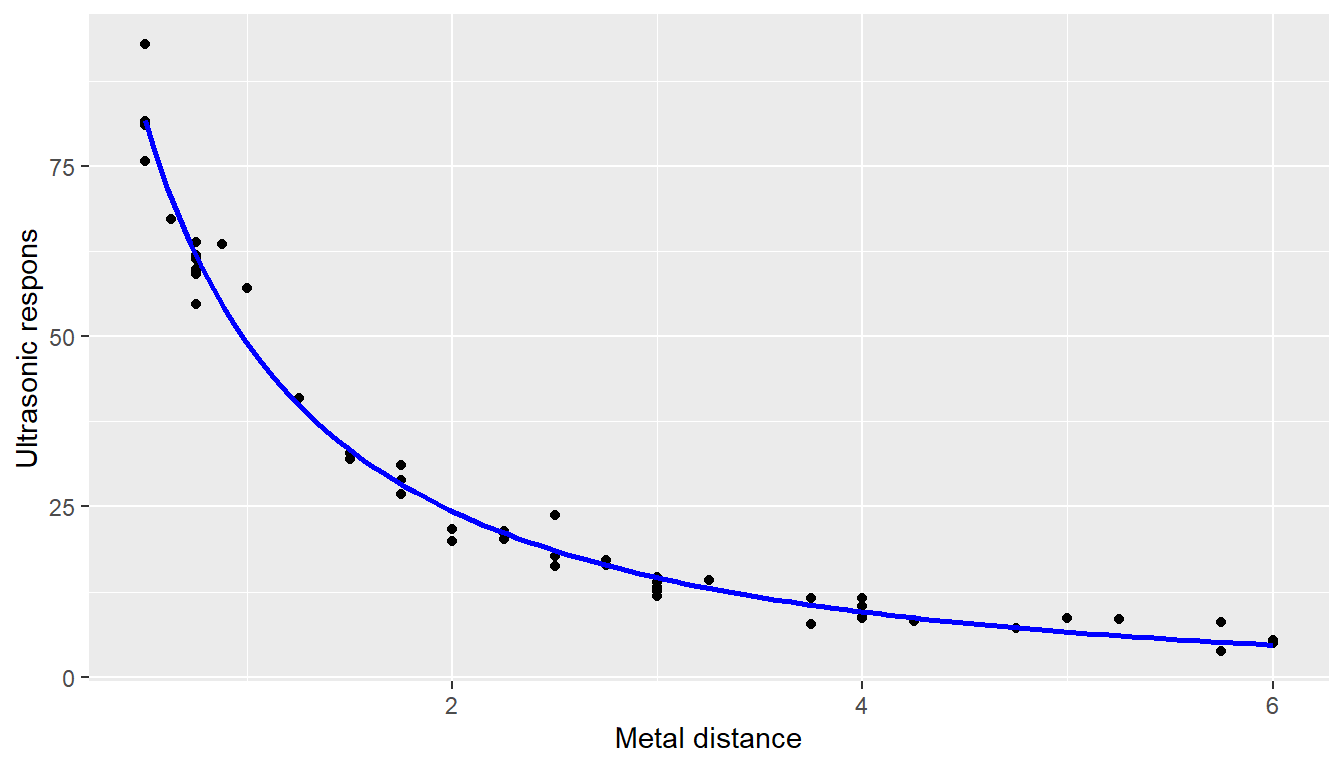# Introduction

## Abstract

• In clinical research the dose–response relationship is often non-linear, therefore advanced fitting models are needed to capture their behavior. The talk will concentrate on fitting non-linear parametric models to the dose–response data and will explain some specificities of this problem.

• The talk is based on the book by Christian Ritz, Jens Carl Streibig “Nonlinear regression with R”.

# Purpose of Dose-Response Information

## ICH E4 Harmonized Tripartite Guideline

Knowledge of the relationships among dose, drug-concentration in blood, and clinical response (effectiveness and undesirable effects) is important for the safe and effective use of drugs in individual patients. It helps identify

• an appropriate starting dose,

• the best way to adjust dosage to the needs of a particular patient,

• a dose beyond which increases would be unlikely to provide added benefit or would produce unacceptable side effects.

## Example

There are other fields of studies were concentration analysis can be used.

Consider the following relationship (Chwirut2 is included in the package NISTnls), where the response variable is ultrasonic response, and the predictor variable is metal distance.

#library(NISTnls)
dim(Chwirut2)
##  54  2
head(Chwirut2)
##         y     x
## 1 92.9000 0.500
## 2 57.1000 1.000
## 3 31.0500 1.750
## 4 11.5875 3.750
## 5  8.0250 5.750
## 6 63.6000 0.875

A distance-amplitude relationship of attenuation that an ultrasound beam experiences traveling through a medium

#library(ggplot2)
g <- ggplot(data=Chwirut2,aes(x=x,y=y)) +
geom_point() + xlab("Metal distance") + ylab("Ultrasonic respons")
g# The built-in function nls()

## Fitting a parametric family

Suppose we observe the following pair of data points $$(x_i,y_i),\,i=1,\cdots,n.$$ Consider a parametric family (usually non-linear) that we want to fit to the data $f(x,(\theta_1,\cdots,\theta_k)).$ The hypothesis is that there is the following relationship between observed points

$y_i=f(x_i,(\theta_1,\cdots,\theta_k))+\varepsilon_i,\ \ i=1,\cdots,n.$

The fitting method that we are going to use is the Non-linear Least Squares (NLS) method. NLS estimates can be found using this minimization problem.

$(\hat\theta_1,\cdots,\hat\theta_k)=\arg\min_{\theta_1,\cdots,\theta_k}\sum_{i=1}^n(y_i-f(x_i,(\theta_1,\cdots,\theta_k)))^2.$

## Gauss - Newton algorithm

The Gauss - Newton algorithm is used to solve the optimization problem described above.

If we denote $$g_i(\theta)=y_i-f(x_i,\theta), \ \ \theta=(\theta_1,\cdots,\theta_k),$$ then the minimization problem is the following $\min_{\theta\in R^k}\sum_{i=1}^ng_i^2(\theta)=\min_{\theta\in R^k}||g(\theta)||^2.$ Choose an initial value $$\theta_0$$ and linearize the function $$g(\theta)$$ around $$\theta_0$$ as follows

$\hat g(\theta,\theta_0)=g(\theta_0)+Dg(\theta_0)(\theta-\theta_0).$ Solving $$\min_{\theta\in R^k}||g(\theta_0)+Dg(\theta_0)(\theta-\theta_0)||^2,$$ where $$m\times n$$ matrix $$Dg(\theta)$$ is the Jacobian, we get $$\theta_1$$ and continuing this process interatively, we get the sequence $$(\theta_m),\,m=0,1,\cdots.$$ Moreover, if $$Dg(\theta_k)$$ has linearly independent columns,

$\theta_{k+1}=\theta_k-\left([Dg(\theta_k)]^TDg(\theta_k)\right)^{-1}[Dg(\theta_k)]^Tg(\theta_k).$ The question is - how to choose the initial value $$\theta_0$$ of iteration?

## The parametric family

The following parametric family was suggested to fit the distance-amplitude relationship

$f(x,(\beta_1,\beta_2,\beta_3))=\frac{\exp(-\beta_1x)}{\beta_2+\beta_3x}$ Assumptions:

1. correct mean function $$f$$

2. variance homogeneity (homoscedasticity)

3. normally distributed measurements errors

4. mutually independent measurement errors $$\varepsilon_i$$

• If we take $$x=0$$ then we get $$f(0,(\beta_1,\beta_2,\beta_3))=\frac{1}{\beta_2}$$ so the initial estimate of $$\beta_2$$ can be the reciprocal of the response value closest to the $$y$$ axis, that is, roughly, $$\frac{1}{100}=0.01.$$ For the

## Fitting non-linear parametric family

expFct <- function(x,beta1,beta2=0.01,beta3)  exp(-beta1*x)/(beta2+beta3*x)
l <- list(beta1=0.1, beta2=0.01, beta3=0.001)
fit <- nls(data=Chwirut2, y ~ expFct(x, beta1, beta2, beta3), start=l)
fit
## Nonlinear regression model
##   model: y ~ expFct(x, beta1, beta2, beta3)
##    data: Chwirut2
##    beta1    beta2    beta3
## 0.166576 0.005165 0.012150
##  residual sum-of-squares: 513
##
## Number of iterations to convergence: 9
## Achieved convergence tolerance: 7.467e-07
tidy(fit)
## # A tibble: 3 × 5
##   term  estimate std.error statistic  p.value
##   <chr>    <dbl>     <dbl>     <dbl>    <dbl>
## 1 beta1  0.167    0.0383        4.35 6.56e- 5
## 2 beta2  0.00517  0.000666      7.75 3.54e-10
## 3 beta3  0.0122   0.00153       7.94 1.81e-10

## Plotting the fitted non-linear function

Hence, we can plot the fitted curve

#library(broom)
K=tidy(fit3)$estimate), colour = "darkgreen")# Defining Self-Starter functions ## A simple example Consider the following parametric family $f(x,(b,y_0))=y_0 e^{\frac{x}{b}}.$ • This model can be used to describe radioactive decay. The parameter $$y_0$$ is the initial amount of the radioactive substance (at time $$x = 0$$). The rate of decay is governed by the second parameter $$b$$ (the inverse decay constant). • The definition of a self-starter contains the formula of the parametric family, a logic of constructing initial values for parameters, a function taking the dataset as argument and other arguments ensuring that the logic of construction of initial values, the definition of parametric family and nls() are tied together. ## The logic of construction of initial values Apply the log transform on the given mean function $\log f(x,(b,y_0))=\log y_0+\frac{x}{b}.$ Hence, if we apply the log transform on the response data, we can apply linear regression techniques to estimate the slope and the intercept, then, from the equalities $\log\tilde y_0={\beta_0}, \ \ \tilde \beta_1=\frac{1}{b}$ we get $\tilde y_0=e^{\tilde \beta_0}, \ \ \tilde b=\frac{1}{\tilde\beta_1}.$ This is the logic of construction of initial parameter values that we need to include in the definition of a Self-Starter. (We are not interested in the error structure that will change after the transformation, because we need only initial estimates.) ## The match.call() function The function match.call() can be used in the definition of the function to store the call in the resulting object. In addition, if that function is called without specifying the arguments, the function match.call() matches the arguments to their names by their positions. Observe below Func <- function(input, parameter){ value <- input^parameter attr(value,"call") <- match.call() value } power <- Func(2,16) #observe that we did not specify the names of parameters power ##  65536 ## attr(,"call") ## Func(input = 2, parameter = 16) Hence, their argument values can be extracted from the call by their names as from a list attr(power,"call")$parameter
##  16

## Three steps

• Define the mean function
expModel <- function(predictor,b, y0) {
y0 * exp(predictor/b)
}
• Define the function with the initialization logic
expModelInit <- function(mCall, LHS, data) {
xy <- sortedXyData(mCall[["predictor"]], LHS, data)
lmFit <- lm(log(xy[, "y"]) ~ xy[, "x"])
coefs <- coef(lmFit)
y0 <- exp(coefs)
b <- 1/coefs
value <- c(b, y0)
names(value) <- c("b","y0")
value
}

# Define the Self-Starter function

## Specificities of working in a Markdown Environment

SSexp <- selfStart(expModel, expModelInit, c("b", "y0"))
class(SSexp)
##  "selfStart"

RStudio actually creates a separate R session to render the document. This causes problem with the getInitial() function which searches for user created SS functions in the Global environment and cannot find them because they are created in a new environment only for the Markdown use. Hence, we need to ensure that our created Self-Starter is defined in the Global environment.

#Only for Markdown execution
l <- list(SSexp = SSexp)
list2env(l, envir = .GlobalEnv)
## <environment: R_GlobalEnv>

## Getting the inital values

Self-starters can be passed as an argument to the function getInitial() to obtain the initial values. Consider the following dataset

load(file = "RGRcurve.rda")
head(RGRcurve)
##   Day   RGR
## 1   0 0.169
## 2   0 0.164
## 3   0 0.210
## 4   0 0.215
## 5   0 0.183
## 6   0 0.181

Then, the initial values, determined according to our logic, will be

getInitial(RGR ~ SSexp(Day, b,y0), data = RGRcurve)
## Warning: selfStart initializing functions should have a final '...' argument
## since R 4.1.0
##         b        y0
## 3.8450187 0.1674235

## Fitting by user defined SS

fit4 <- nls(RGR ~ SSexp(Day, b,y0), data = RGRcurve)
## Warning: selfStart initializing functions should have a final '...' argument
## since R 4.1.0
getInitial(RGR ~ SSexp(Day, b,y0), data = RGRcurve)
## Warning: selfStart initializing functions should have a final '...' argument
## since R 4.1.0
##         b        y0
## 3.8450187 0.1674235
# Compare the initial values with the estimated values
tidy(fit4)
## # A tibble: 2 × 5
##   term  estimate std.error statistic  p.value
##   <chr>    <dbl>     <dbl>     <dbl>    <dbl>
## 1 b        3.76     0.175       21.5 3.40e-23
## 2 y0       0.164    0.0142      11.5 4.03e-14

g <- ggplot(data = RGRcurve, aes(x = Day, y = RGR)) + geom_point()
g + stat_function(fun = SSexp, args=tidy(fit4)$estimate, colour="darkgreen")# Difficult Example ## Returning to the difficult example Considering once again the parametric family $f(x,\beta)=\frac{\exp(-\beta_1x)}{\beta_2+\beta_3x},\ \ \beta=(\beta_1,\beta_2,\beta_3).$ We can use Taylor’s formula $f(x,\beta)\approx f(0,\beta)+f'(0,\beta)x,\ \ x\approx 0.$ Simplifying this and remembering that $$f(0,\beta)=\frac{1}{\beta_2}$$ we get $f(x,\beta)\approx \frac{1}{\beta_2}-\frac{1}{\beta_2}\left(\beta_1+\frac{\beta_3}{\beta_2}\right)x.$ Which in turn gives the equality $1-\beta_2 f(x,\beta)=\left(\beta_1+\frac{\beta_3}{\beta_2}\right)x,$ hence, transforming the data according to the left hand side and fitting a linear model without an intercept we can estimate $$\left(\beta_1+\frac{\beta_3}{\beta_2}\right).$$ For two parameters out of three we have a rule to find starting values, for the third one we still need a guess. Taking $$\beta_1=0$$ may work. expFctInit <- function(mCall, LHS, data){ xy <- sortedXyData(mCall[["x"]], LHS, data) beta2 <- 1/xy[1,"y"] coefs <- coef(lm(1-beta2*xy[,"y"]~xy[,"x"]+0)) beta1 <- 0 beta3 <- coefs*beta2 value <- c(beta1, beta2, beta3) names(value) <- c("beta1", "beta2", "beta3") value } SSexpFct <- selfStart(expFct, expFctInit, c("beta1", "beta2", "beta3")) l <- list(SSexpFct=SSexpFct) list2env(l, envir = .GlobalEnv)#Only for Markdown execution ## <environment: R_GlobalEnv> getInitial(data = Chwirut2, y ~ SSexpFct(x, beta1, beta2, beta3)) ## Warning: selfStart initializing functions should have a final '...' argument ## since R 4.1.0 ## beta1 beta2 beta3 ## 0.000000000 0.012140834 0.002537341 fit0 <- nls(data = Chwirut2, y ~ SSexpFct(x, beta1, beta2, beta3)) ## Warning: selfStart initializing functions should have a final '...' argument ## since R 4.1.0 fit0_tidy <- tidy(fit0) fit0_tidy$p.value <- format.pval(fit0_tidy$p.value,eps=0.001,digits=3) CI <- as.data.frame(suppressMessages(confint(fit0))) CI$term <- row.names(CI)
fit0_tidy <- merge(fit0_tidy,CI,by="term")
fit0_tidy[,!names(fit0_tidy)%in%c("term", "p.value")]<-
round(fit0_tidy[,!names(fit0_tidy)%in%c("term", "p.value")], 3)
fit0_tidy
##    term estimate std.error statistic p.value  2.5% 97.5%
## 1 beta1    0.167     0.038     4.349  <0.001 0.094 0.254
## 2 beta2    0.005     0.001     7.753  <0.001 0.004 0.006
## 3 beta3    0.012     0.002     7.939  <0.001 0.009 0.015

Here, we used the generic function $$confint()$$ on the object of class $$nls$$ and got the confidence intervals for the parameters estimates. In addition, the function $$format.pval()$$ was used to simplify the presentation of p.values.

values <- tidy(fit0)\$estimate
g <- ggplot(data = Chwirut2, aes(x = x, y = y))+
geom_point() + xlab("Metal distance") + ylab("Ultrasonic respons")
g + stat_function(fun = expFct, args = values,
colour="blue", lwd=1)predict(fit0, newdata = data.frame(x=6:7))
##  4.715006 3.453957

• The package drc (Dose-Response curves) contains much more prespecified parametric families (22, to be exact - run drc::getMeanFunctions() to see the list).

• The package nlme (Linear and Nonlinear Mixed Effects Models) which allows the inclusion of the donor effect as a random effect in dose-response studies.

• More robust algorithms for fitting (The Levenberg-Marquardt curve-fitting method)

## The Levenberg-Marquardt method

• The Levenberg-Marquardt curve-fitting method is a combination of the two other minimization methods: the gradient descent method and the Gauss-Newton method.

• In the gradient descent method, the sum of the squared errors is reduced by updating the parameters in the steepest-descent direction.

• In the Gauss-Newton method, the sum of the squared errors is reduced by assuming the least squares function is locally quadratic, and finding the minimum of the quadratic.

• The Levenberg-Marquardt method acts more like a gradient-descent method when the parameters are far from their optimal value, and acts more like the Gauss-Newton method when the parameters are close to their optimal value.

• Henri P. Gavin, The Levenberg-Marquardt method for nonlinear least squares curve-fitting problems

# Presentation

Below is a presentation version of this lecture which contains also interactive applications for curve fitting.##### Samvel B. Gasparyan
###### Biostatistician

Biostatistician in cardiovascular trials.